Anzeige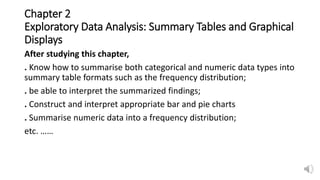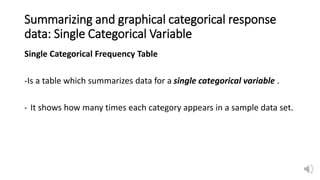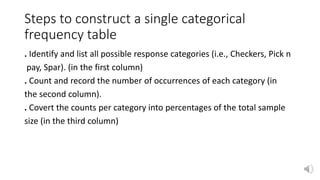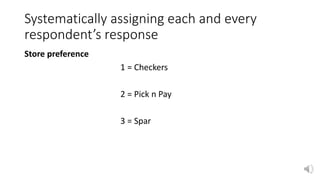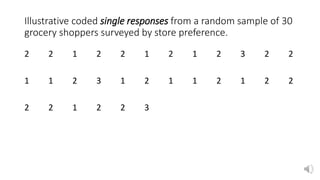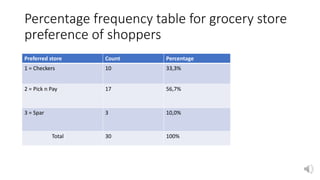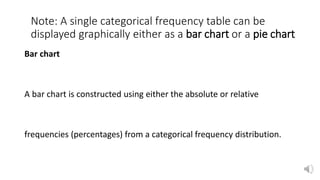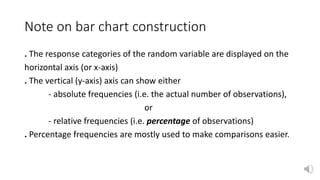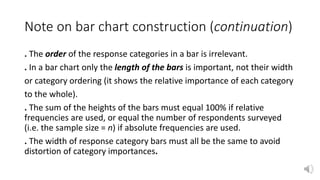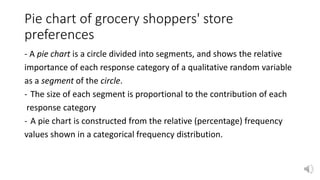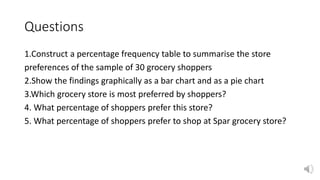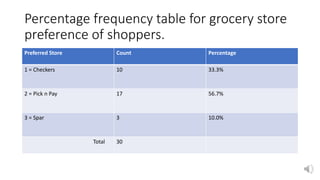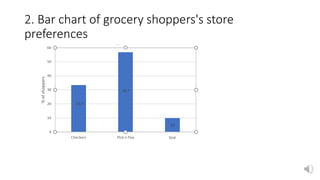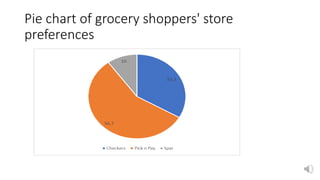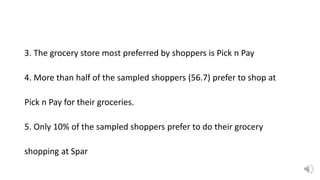1 von 15
Anzeige

### Chapter 2 @ Exploratory Data Analysis -1.pptx

1. Chapter 2 Exploratory Data Analysis: Summary Tables and Graphical Displays After studying this chapter, . Know how to summarise both categorical and numeric data types into summary table formats such as the frequency distribution; . be able to interpret the summarized findings; . Construct and interpret appropriate bar and pie charts . Summarise numeric data into a frequency distribution; etc. ……
2. Summarizing and graphical categorical response data: Single Categorical Variable Single Categorical Frequency Table -Is a table which summarizes data for a single categorical variable . - It shows how many times each category appears in a sample data set.
3. Steps to construct a single categorical frequency table . Identify and list all possible response categories (i.e., Checkers, Pick n pay, Spar). (in the first column) . Count and record the number of occurrences of each category (in the second column). . Covert the counts per category into percentages of the total sample size (in the third column)
4. Systematically assigning each and every respondent’s response Store preference 1 = Checkers 2 = Pick n Pay 3 = Spar
5. Illustrative coded single responses from a random sample of 30 grocery shoppers surveyed by store preference. 2 2 1 2 2 1 2 1 2 3 2 2 1 1 2 3 1 2 1 1 2 1 2 2 2 2 1 2 2 3
6. Percentage frequency table for grocery store preference of shoppers Preferred store Count Percentage 1 = Checkers 10 33,3% 2 = Pick n Pay 17 56,7% 3 = Spar 3 10,0% Total 30 100%
7. Note: A single categorical frequency table can be displayed graphically either as a bar chart or a pie chart Bar chart A bar chart is constructed using either the absolute or relative frequencies (percentages) from a categorical frequency distribution.
8. Note on bar chart construction . The response categories of the random variable are displayed on the horizontal axis (or x-axis) . The vertical (y-axis) axis can show either - absolute frequencies (i.e. the actual number of observations), or - relative frequencies (i.e. percentage of observations) . Percentage frequencies are mostly used to make comparisons easier.
9. Note on bar chart construction (continuation) . The order of the response categories in a bar is irrelevant. . In a bar chart only the length of the bars is important, not their width or category ordering (it shows the relative importance of each category to the whole). . The sum of the heights of the bars must equal 100% if relative frequencies are used, or equal the number of respondents surveyed (i.e. the sample size = n) if absolute frequencies are used. . The width of response category bars must all be the same to avoid distortion of category importances.
10. Pie chart of grocery shoppers' store preferences - A pie chart is a circle divided into segments, and shows the relative importance of each response category of a qualitative random variable as a segment of the circle. - The size of each segment is proportional to the contribution of each response category - A pie chart is constructed from the relative (percentage) frequency values shown in a categorical frequency distribution.
11. Questions 1.Construct a percentage frequency table to summarise the store preferences of the sample of 30 grocery shoppers 2.Show the findings graphically as a bar chart and as a pie chart 3.Which grocery store is most preferred by shoppers? 4. What percentage of shoppers prefer this store? 5. What percentage of shoppers prefer to shop at Spar grocery store?
12. Percentage frequency table for grocery store preference of shoppers. Preferred Store Count Percentage 1 = Checkers 10 33.3% 2 = Pick n Pay 17 56.7% 3 = Spar 3 10.0% Total 30
13. 2. Bar chart of grocery shoppers's store preferences
14. Pie chart of grocery shoppers' store preferences
15. 3. The grocery store most preferred by shoppers is Pick n Pay 4. More than half of the sampled shoppers (56.7) prefer to shop at Pick n Pay for their groceries. 5. Only 10% of the sampled shoppers prefer to do their grocery shopping at Spar
Anzeige